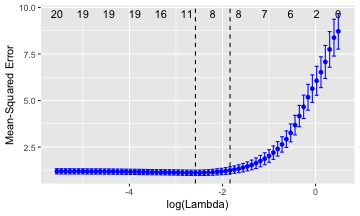This document explains plotting diagnostics for LM and GLM using `{ggplot2}` and `{ggfortify}`.

# Plotting Diagnostics for Linear Models

`{ggfortify}` let `{ggplot2}` know how to interpret `lm` objects. After loading `{ggfortify}`, you can use `ggplot2::autoplot` function for `lm` objects.

``````library(ggfortify)
autoplot(lm(Petal.Width ~ Petal.Length, data = iris), label.size = 3)
``````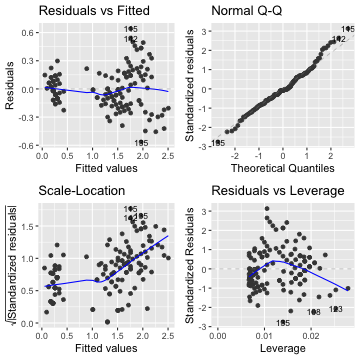You can select desired plot by `which` option as the same manner as standard `plot`. Also, `ncol` and `nrow` allows you to specify the number of subplot columns and rows.

``````par(mfrow = c(1, 2))
m <- lm(Petal.Width ~ Petal.Length, data = iris)

autoplot(m, which = 1:6, ncol = 3, label.size = 3)
``````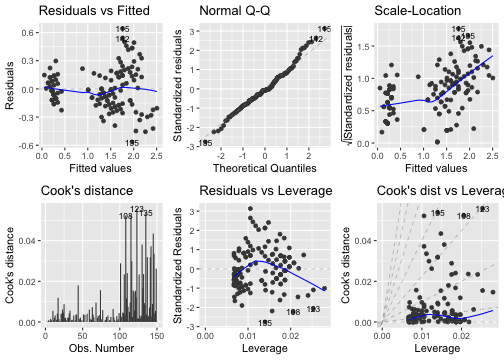# Plotting Diagnostics for Generalized Linear Models

It also suppotgs `glm` instance.

``````m <- glm(Murder ~ Assault + UrbanPop + Rape,
family = gaussian, data = USArrests)

autoplot(m, which = 1:6, label.size = 3)
``````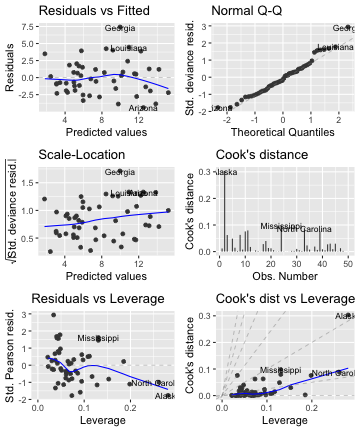# Decorating Plots

Because `{ggplot2}` itself cannot handle different kinds of plots in a single instance, `{ggfortify}` handle them using its original class named `ggmultiplot`. You can use `+` operator to decorate `ggmultiplot`.

``````class(autoplot(m))
``````
``````##  "ggmultiplot"
## attr(,"package")
##  "ggfortify"
``````
``````autoplot(m, label.size = 3) + theme_bw()
``````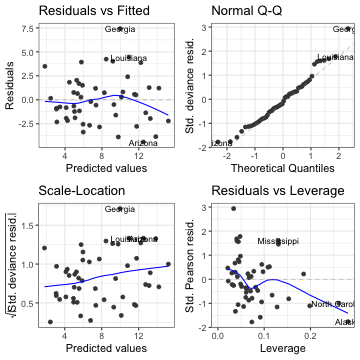# Specifing Plotting Options

Some properties can be changed by passing corresponding keywords. For example, `colour` keyword is for data points, `smooth.colour` is for smoothing lines and `ad.colour` is for additional auxiliary lies. Also, `ncol` and `nrow` control facet layout. Use `help(autoplot.lm)` (or `help(autoplot.*)` for any other objects) to check available options.

``````autoplot(m, which = 1:6, colour = 'dodgerblue3',
smooth.colour = 'black', smooth.linetype = 'dashed',
label.size = 3, label.n = 5, label.colour = 'blue',
ncol = 3)
``````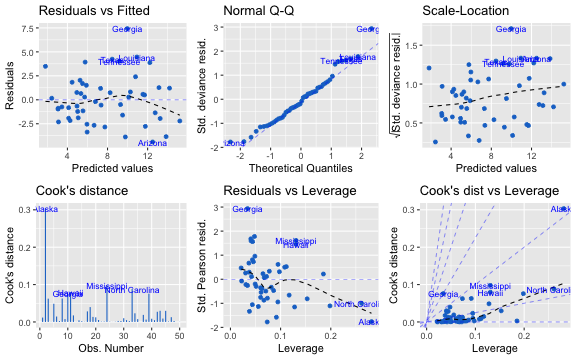Also, you can use column names for these properties. Note that `lm` and `glm` instances doesn't retain original data, you should pass original data via `data` keyword to use column names not included in the model.

``````autoplot(lm(Petal.Width ~ Petal.Length, data = iris), data = iris,
colour = 'Species', label.size = 3)
``````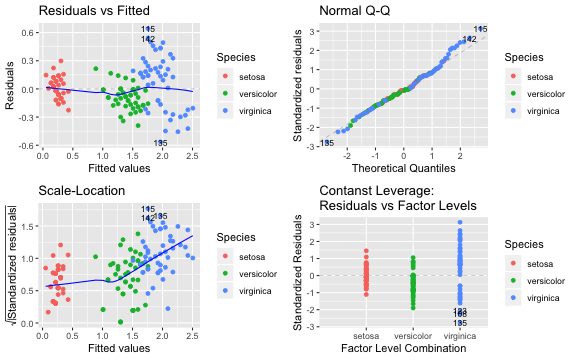# Plotting `{glmnet}`

`{ggfortify}` supports `{glmnet}` package which supports Regularized Generalized Linear Models (Ridge, Lasso and Elastic-net).

``````library(glmnet)
data(QuickStartExample)
fit <- glmnet::glmnet(x, y)
autoplot(fit)
``````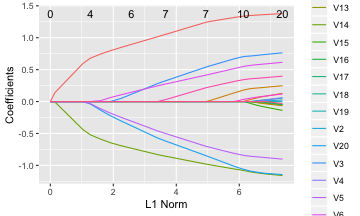``````fit <- glmnet::cv.glmnet(x, y)
autoplot(fit, colour = 'blue')
``````